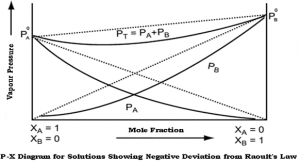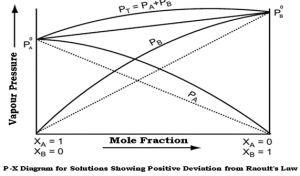# Solubility: Ideal and non ideal solutions and MCQs for GPAT, NIPER, Pharmacist and Drug Inspector exam

## Solubility: Ideal and non ideal solutions and MCQs for GPAT, NIPER, Pharmacist and Drug Inspector exam

Ideal and Real Solutions:

Ideal solution:

• No change in the properties of the components, other than dilution, when they are mixed to form the solution.
• No heat is evolved or absorbed during the mixing process,
• The final volume of the solution represents an additive property of the individual constituents. no shrinkage or expansion occurs when the substances are mixed.
• The constitutive properties, for example, the vapor pressure, refractive index, surface tension, and viscosity of the solution, are the weighted averages of the properties of the pure individual constituents.
• Mixing substances with similar properties forms ideal solutions.
• When 100 mL of methanol is mixed with 100 mL of ethanol, the final volume of the solution is 200 mL, and no heat is evolved or absorbed. The solution is nearly ideal.
• When 100 mL of sulfuric acid is combined with 100 mL of water, the volume of the solution is about 180 mL at room temperature, and the mixing is attended by a considerable evolution of heat; the solution is said to be non ideal, or real.

Ideal Solutions and Raoult’s Law:

In an ideal solution, the partial vapor pressure of each volatile constituent is equal to the vapor pressure of the pure constituent multiplied by its mole fraction in the solution. Thus, for two constituents A and B,

P = Pa + Pb

Pa = PaºXa

Pb = PbºXb

Real Solutions:

• Ideality in solutions presupposes complete uniformity of attractive forces.
• Many examples of solution pairs are known, however, in which the “cohesive” attraction of A for A exceeds the “adhesive” attraction existing between A and B. Similarly, the attractive forces between A and B may be greater than those between A and A or B and B.
• Such mixtures are real or nonideal;
• Real solutions do not adhere to Raoult’s law throughout the entire range of composition.

Two types of deviation from Raoult’s law:

1. Negative deviation.
2. Positive deviation.

Negative deviation:

• When the “adhesive” attractions between molecules of different species exceed the “cohesive” attractions between like molecules,
• The vapor pressure of the solution is less than that expected from Raoult’s ideal solution law,
• Example: chloroform and acetone.Fig 1 – Diagram showing negative deviation from Raoult’s law(taken from NCERT Chemistry Part 1)

Positive deviation:

• When the interaction between A and B molecules is less than that between molecules of the pure constituents,
• The partial vapor pressure of the constituents is greater than that expected from Raoult’s law,
• Examples: benzene and ethyl alcohol, chloroform and ethyl alcohol.Fig 2 – Diagram showing positive deviation from Raoult’s law(taken from NCERT Chemistry Part 1)

In real solutions, the behavior of the component present in high concentration (the solvent) can be described by Raoult’s law as:

Psolvent = solventXsolvent

It cannot hold for the component in low concentration (the solute).

Multiple choice questions(MCQs)

1.Which of the following statements regarding Ideal solutions is false?

a)Ideal solutions obey Raoult’s law under all conditions of temperature and concentrations

b)There will be some change in volume on mixing the components, i.e., ΔVmixing≠ 0

c)There will be no change in enthalpy when the two components are mixed, i.e., ΔHmixing = 0

d)There will be no change in volume on mixing the components, i.e., ΔVmixing= 0

2.Which of the following is not an example of an Ideal solution?

a)Benzene + Toluene

b)n-Hexane + n-Heptane

c)Ethyl alcohol + Water

d)Ethyl bromide + Ethyl chloride

3.A solution which does not obey Raoult’s law is called a non-ideal solution.

a)True

b)False

4.Which of the following is false regarding Non-Ideal solutions?

a)They do not obey Raoult’s law

b)ΔVmixing≠ 0

c)ΔHmixing = 0

d)They form azeotropes

5.Which of the following is an example of a non-ideal solution showing positive deviation?

a)Acetone + Carbon disulphide

b)Chlorobenzene + Bromobenzene

c)Chloroform + Benzene

d)Acetone + Aniline

6.Ideal solutions do not form azeotropes.

a)True

b)False

7.Which of the following is not an example of a non-ideal solution showing negative deviation?

a)HNO3 + Water

b)HCl + Water

c)Acetic acid + Pyridine

d)Carbon tetrachloride + Toluene

8.Which of the following is true regarding non-ideal solutions with negative deviation?

a)The interactions between the components are lesser than in the pure components

b)ΔVmixing= +ve

c)ΔHmixing= +ve

d)They form maximum boiling azeotrope

9.Which of the following cannot form an azeotrope?

a)H2O + C2H5OH

b)CHCl3+ C2H5OH

c)HCl + H2O

d)Benzene + Toluene

10.Which of the following is true regarding azeotropes?

a)An azeotrope does not exhibit the same concentration in the vapour phase and the liquid phase

b)Azeotropic mixtures cannot be separated into their constituents by fractional distillation

c)In case of minimum boiling azeotropes, the boiling point of the azeotrope is higher than the boiling point of either of the pure components

d)In case of maximum boiling azeotropes, the boiling point of the azeotrope is lesser than the boiling point of either of the pure components

11.If liquids A and B form an ideal solution, then what is the Gibbs free energy of mixing?

a)> 0

b)< 0

c)= 0

d)Not Defined

12.5 moles of liquid X and 10 moles of liquid Y make a solution having a total vapour pressure 70 torr. The vapour pressures of pure X and pure Y are 64 torr and 76 torr respectively. Which of the following is true regarding the described solution?

a)The solution shows positive deviation

b)The solution shows negative deviation

c)The solution is ideal

d)The solution has volume greater than the sum of individual volumes

13.Solutions which show positive or negative deviation from the Raoult’s law are called

a)Ideal solutions

b)True solutions

c)Non ideal solutions

d)Homogenous solutions

14.Which of the following components form an ideal solution?

a)Ethyl alcohol and benzene

b)Acetone and aniline

c)Water and nitric acid

d)Benzene and toluene

15.According to Raoult’s law the partial vapour pressure of each component in the solution is directly proportional to its

a)Volume

b)Mole fraction

c)Molality

d)Density

Solutions:

1. b)There will be some change in volume on mixing the components, i.e., ΔVmixing ≠ 0
2. c)Ethyl alcohol + Water
3. a)True
4. c)ΔHmixing = 0
5. a)Acetone + Carbon disulphide
6. a)True
7. d)Carbon tetrachloride + Toluene
8. d)They form maximum boiling azeotrope
9. d)Benzene + Toluene
10. b)Azeotropic mixtures cannot be separated into their constituents by fractional distillation
11. b)< 0
12. b)The solution shows negative deviation
13. c)Non ideal solutions
14. d)Benzene and toluene
15. b)Mole fraction

References:

1. Martins Physical Pharmacy, 6th edition 2011, page no. 332-355.

For More Standard and Quality Question Bank you can Join Our Test Series Programme for GPAT, NIPER JEE, Pharmacist Recruitment Exam, Drug Inspector Recruitment Exams, PhD Entrance Exam for Pharmacy

List of Successful GPATINDIAN CANDIDATES

Participate in CSIR NET JRF Mock Test

Participate GATE Mock Test

×
Free Video Lectures of Pharmacy Exams
Apply now

Developed By Connect Globes